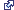# Porous Puff (Spatial Analyst)

## Summary

Calculates the time-dependent, two-dimensional concentration distribution in mass per volume of a solute introduced instantaneously and at a discrete point into a vertically mixed aquifer.

## Usage

• The effective porosity field, a physical property of the aquifer, is generally estimated from geological data. It is defined as the volume of void space that contributes to fluid flow divided by the entire volume. Porosity is expressed as a number between 0.0 and 1.0, with typical values around 0.35, and is dimensionless. A value of effective porosity of 0.35 means that 35 percent of the volume of the porous medium contributes to fluid flow. The remaining 65 percent, consisting of solid matrix and unconnected pores, does not contribute to fluid flow.

• No particular system of units is specified by this tool. It is important that all data be consistent, using the same unit for time (seconds, days, years), length (feet, meters), and mass (kilograms, slugs).

• The saturated thickness, measured in units of length, is interpreted from geological information. For a confined aquifer, this measure is the thickness of the formation between the upper and lower confining layers. For an unconfined aquifer, the saturated thickness is the distance between the water table and the lower confining layer.

• The decay coefficient λ is related to the half-life T1/2 as:

For example, the half-life of Carbon-14 is 5,730 years. Since ln(2) = 0.693, the decay coefficient becomes 0.693/5730 = 1.21x10-4 /year. A stable constituent has a decay coefficient of zero, corresponding to an infinite half-life. Half-lives of radioisotopes are available from several sources, including the CRC Handbook of Chemistry and Physics from CRC Press.

• The requested time must not exceed the latest time recorded in the path file. Either a lesser time must be requested in Porous Puff, or a new path file with a greater time needs to be generated by Particle Track.

• The time requested should not be reached before the completion of the first path step as recorded in the track file. Either a greater time should be requested in Porous Puff, or a new track file should be generated by Particle Track using a shorter step length.

• The centroid of mass must not migrate to the edge of the raster or beyond. In this case, no data is available on which to base the dispersion, so the tool is aborted. Either a lesser time must be requested, or a larger raster must be generated to accommodate the migration.

• The output raster is floating point.

• See Analysis environments and Spatial Analyst for additional details on the geoprocessing environments that apply to this tool.

## Syntax

`PorousPuff(in_track_file, in_porosity_raster, in_thickness_raster, mass, {dispersion_time}, {longitudinal_dispersivity}, {dispersivity_ratio}, {retardation_factor}, {decay_coefficient})`
 Parameter Explanation Data Type in_track_file The input particle track path file.This is an ASCII text file containing information about the position, the local velocity vector, and the cumulative length and time of travel along the path.This file is generated using the Particle Track tool. File in_porosity_raster The input raster where each cell value represents the effective formation porosity at that location. Raster Layer in_thickness_raster The input raster where each cell value represents the saturated thickness at that location.The value for the thickness is interpreted from geological properties of the aquifer. Raster Layer mass A value for the amount of mass released instantaneously at the source point, in units of mass. Double dispersion_time(Optional) A value representing the time horizon for dispersion of the solute, in units of time.The time must be less than or equal to the maximum time in the track file. If the requested time exceeds the available time from the track file, the tool is aborted. The default time is the latest time (corresponding to the terminal point) in the track file. Double longitudinal_dispersivity(Optional) A value representing the dispersivity parallel to the flow direction.For details on how the default value is determined, and how it relates to the scale of the study, see the How Porous Puff works section in the documentation. Double dispersivity_ratio(Optional) A value representing the ratio of longitudinal dispersivity over transverse dispersivity.Transverse dispersivity is perpendicular to the flow direction in the same horizontal plane. The default value is three. Double retardation_factor(Optional) A dimensionless value representing the retardation of the solute in the aquifer.Retardation varies between one and infinity, with one corresponding to no retardation. The default value is one. Double decay_coefficient(Optional) Decay coefficient for solutes undergoing first-order exponential decay (for example, radionuclides) in units of inverse time.The default is zero, corresponding to no decay. Double

#### Return Value

 Name Explanation Data Type out_raster The output raster of the concentration distribution.Each cell value represents the concentration at that location. Raster

## Code sample

PorousPuff example 1 (Python window)

This example executes the tool on the required inputs and outputs a raster of the concentration distribution.

``````import arcpy
from arcpy import env
from arcpy.sa import *
env.workspace = "C:/sapyexamples/data"
outPorousPuff = PorousPuff("trackfile.txt", "gwporo", "gwthick", 50, 10000, "", 3,
"", "")
outPorousPuff.save("c:/sapyexamples/output/outporpuff")``````
PorousPuff example 2 (stand-alone script)

This example executes the tool on the required inputs and outputs a raster of the concentration distribution.

``````# Name: PorousPuff_Ex_02.py
# Description: Calculates the time-dependent, two-dimensional
#              concentration distribution in mass per volume of a
#              solute introduced instantaneously and at a discrete
#              point into a vertically mixed aquifer.

# Requirements: Spatial Analyst Extension

# Import system modules
import arcpy
from arcpy import env
from arcpy.sa import *

# Set environment settings
env.workspace = "C:/sapyexamples/data"

# Set local variables
inTrackFile = "trackfile.txt"
inPorosityRaster = "gwporo"
inThicknessRaster = "gwthick"
mass = 50
dispersionTime = 10000
longitudinalDispersivity = ""
dispersivityRatio = 3
retardationFactor = ""
decayCoefficient = 0

# Execute PorousPuff
outPorousPuff = PorousPuff(inTrackFile, inPorosityRaster, inThicknessRaster,
mass, dispersionTime, longitudinalDispersivity,
dispersivityRatio, retardationFactor,
decayCoefficient)

# Save the output
outPorousPuff.save("c:/sapyexamples/output/outporpuff")``````

## Licensing information

• Basic: Requires Spatial Analyst
• Standard: Requires Spatial Analyst